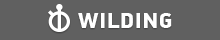GMAT Question of the Day - Daily to your Mailbox; hard ones only

 It is currently 26 Jun 2019, 11:19GMAT Club Daily Prep

Thank you for using the timer - this advanced tool can estimate your performance and suggest more practice questions. We have subscribed you to Daily Prep Questions via email.

Customized
for You

we will pick new questions that match your level based on your Timer History

Track

every week, we’ll send you an estimated GMAT score based on your performance

Practice
Pays

we will pick new questions that match your level based on your Timer History

Not interested in getting valuable practice questions and articles delivered to your email? No problem, unsubscribe here.If the range of the six positive integers 4,3,14,7,10 and x is 14

Author Message
TAGS:

Hide Tags

Senior ManagerD
Status: Manager
Joined: 27 Oct 2018
Posts: 365
Location: Egypt
GPA: 3.67
WE: Pharmaceuticals (Health Care)
If the range of the six positive integers 4,3,14,7,10 and x is 14  [#permalink]

Show Tags

400:00

Difficulty:95% (hard)

Question Stats:22% (02:03) correct78% (01:21) wrongbased on 52 sessions

HideShow timer StatisticsIf the range of the six positive numbers 4,3,14,7,10 and x is 14, what is the difference between the greatest possible value of x and least possible value of x?

(A) 0
(B) 11
(C) 14
(D) 17
(E) 20

_________________
Math ExpertV
Joined: 02 Aug 2009
Posts: 7765
If the range of the six positive integers 4,3,14,7,10 and x is 14  [#permalink]

Show Tags

Mahmoudfawzy83 wrote:
If the range of the six positive numbers 4,3,14,7,10 and x is 14, what is the difference between the greatest possible value of x and least possible value of x?

(A) 0
(B) 11
(C) 14
(D) 17
(E) 20

For all those who have answered wrong, the importance of reading the question for minor details is very important, as POSITIVE word here
The numbers can be written in an ascending order as 3,4,7,10, and 14, and X can fit in somewhere in the series..
Now the range is 14, means difference between the largest and smallest term is 14
As the range without x is 14-3=11, x will be either the largest or smallest
1) let 14 be the highest then smallest will be 14 - 14=0.
This means x is 0, but it is given that X is positive number so X>0.... Not possible
2) let 3 be the minimum, then largest will be 3+14=17
So X is 17..

Thus, the only possible way the range is 14 is 3, 4, 7, 10, 14, 17
So X can be only 17..
Minimum value of x = Maximum value of x=17

A
_________________
e-GMAT RepresentativeD
Joined: 04 Jan 2015
Posts: 2909
Re: If the range of the six positive integers 4,3,14,7,10 and x is 14  [#permalink]

Show Tags

Solution

Given:
• Range of six positive i=numbers, {4, 3, 14, 7, 10, x} = 14

To find:
• The difference between maximum and minimum possible values of x

Approach and Working:
• If x > 14, then x is the maximum value of the set
• And, range will be x – 3 = 14
o Implies, x = 17

• If x < 14, then 14 is the maximum value of the set
• And, for x to be minimum, x must be the minimum value of the set. So, range will be 14 – x = 14
o Implies, x = 0
o But we know x is positive. So, this case is not valid

Thus, x can take only one value = 17

• Therefore, maximum value – minimum value = 17 – 17 = 0

Hence, the correct answer is Option A

_________________Re: If the range of the six positive integers 4,3,14,7,10 and x is 14   [#permalink] 02 Jan 2019, 04:19
Display posts from previous: Sort by

If the range of the six positive integers 4,3,14,7,10 and x is 14